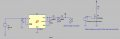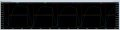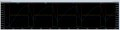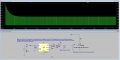# IR LED pulsing with capacitors for current limited battery

#### leonhart88

Joined Feb 23, 2007
118
Hi All,

I'm tackling an interesting problem. I have a current limited battery source (for intrinsic safety) which is limited to 250mA. This battery can either be 3V or 6V. If I can get it working with 3V then that would be ideal. The problem is that I need to pulse an IR LED with 600mA for 5us every 26us in order to transmit data. The source is limited to 250mA by a series resistor. This resistor causes issues due to undesirable voltage drops. I want to use capacitors to provide the extra current, but I also need to stay under 100uF for intrinsic safety requirements.

Assuming our source voltage is 6V and we regulate it down to 3V, I've done some very crude calculations to try and determine how much capacitance is required. We know our required energy, E = (600mA*3V)*5us = 10uJ. And using E = 1/2*C*V^2, I can find the capacitance required, which is around 2uF. Since I don't want to use all the energy in the capacitor in one shot, we can use 20uF for a 10% loss of energy in each pulse. I am not confident that this is the right way to calculate things.

I did some simulations in LTSpice and was getting confusing results, especially when there was a series resistor put inline with the source. It seems that in every configuration, the current being drawn from the regulator is too large and I end up getting huge voltage drops across the source resistor which ends up messing everything up.

I then proceeded to simulate without a series resistor to see how I can lower the current draw from the regulator (thus the source), and shift current output more towards the capacitance. See the pictures below for the schematic and the test outputs. Red is the current going through R3 (I did not have a SPICE model of the LED, so I just used the resistor as the load), blue is the current leaving the regulator (R4), yellow is the current leaving R5, and white or green is the voltage across the capacitor C2.

Using a capacitance of 100uF (C2), I can reduce the current from the regulator by almost two fold compared to using a 30uF capacitor. However, this current will still cause issues with a series resistor.

Does anyone have some advice on the circuit and also the theory on how to calculate these things out? I'd like to be able to calculate things out to determine if this is even possible. What I'm particularly interested in, is how I can manipulate the circuit to minimize current from the regulator and move most of the current output to the capacitors so that I do not cause a large voltage drop on the source. Should I also put a current limiting resistor on the regulator output side? I assume this will effect my capacitor charging time.

Any help would be greatly appreciated, thanks everyone!#### ericgibbs

Joined Jan 29, 2010
18,283
hi leon,
Perhaps I am missing something in your description, but why does the LED have to be powered from the 3Vout.?

Have you considered using the Vsrc, 3v or 5v to power the LED circuit.
An IR emitter diode forward voltage drop is approx 1.8V, so calculate the value of the LED's series resistor.
eg: if the LED required say 20mA, then the series resistor would be [5V-1.8v]/ 0.02 = ~150R
E

#### Bernard

Joined Aug 7, 2008
5,784
Why not use the full 6 V & 100 uF cap. with a switched constant current ckt. to supply the 5 us 600 mA pulse?

#### ronv

Joined Nov 12, 2008
3,770
If I understand correctly this should work. The possible problem would be that the 6 volt supply will be in current limit on power up to initially charge the cap. Don't know if that's a problem for you or not.
The 2N2222 will be a little toasty. (65C) If that's to hot you can use a larger package transistor.

#### Attachments

•Bernard

#### leonhart88

Joined Feb 23, 2007
118
hi leon,
Perhaps I am missing something in your description, but why does the LED have to be powered from the 3Vout.?

Have you considered using the Vsrc, 3v or 5v to power the LED circuit.
An IR emitter diode forward voltage drop is approx 1.8V, so calculate the value of the LED's series resistor.
eg: if the LED required say 20mA, then the series resistor would be [5V-1.8v]/ 0.02 = ~150R
E
The LED does not have to be powered from the 3V out. I just thought this would be more controlled to use the regulated voltage, as the battery can vary with discharge and also with temperature. I simulated it using 6V as the source and a 30uF cap on the input side and it seems to work better, as the capacitors can store more energy with the higher voltage. However, as the voltage can go from 6V to 4V, I'm not sure if I can rely on this method. Also, the IR LED will only be pulsing for a couple of seconds once a button is pushed and will remain off otherwise. Therefore, when the IR LED isn't on, the voltage across the storage capacitor is quite high, which causes the very large pulses of current you see in the beginning. The IR LED may not be able to handle these peak currents, and even if it could, I think that would be undesirable.If I understand correctly this should work. The possible problem would be that the 6 volt supply will be in current limit on power up to initially charge the cap. Don't know if that's a problem for you or not.
The 2N2222 will be a little toasty. (65C) If that's to hot you can use a larger package transistor.
I'll take a close look at this circuit to try and understand what's going on today. One issue is that the series resistor is likely to be quite higher than 5 ohms. If I have to limit the current to 250mA, that resistor is going to be around 24ohms and that voltage drop may effect the rest of my circuitry.

With respect to everyone else's comments, I will do some research into constant current sources (I'm not that familiar with them) and come back with any questions I have. Thanks for the input everyone!

#### ronv

Joined Nov 12, 2008
3,770
The five ohms is only to limit the charge current. The 2 transistors set the LED current at 600ma.
Is the plan to run other stuff from power after the resistor?
If so you might want a separate voltage regulator run off the 6 volt supply to power them as the voltage after the resistor bounces around a lot.

#### leonhart88

Joined Feb 23, 2007
118
The five ohms is only to limit the charge current. The 2 transistors set the LED current at 600ma.
Is the plan to run other stuff from power after the resistor?
If so you might want a separate voltage regulator run off the 6 volt supply to power them as the voltage after the resistor bounces around a lot.
Ah I see. Yes, there will be roughly 40-50mA required on average for other circuitry like indicator LEDs, microcontroller, etc.

#### ronv

Joined Nov 12, 2008
3,770
Here is the spice file.

#### Attachments

• 1.8 KB Views: 21